# Plotting Earth relief¶

Plotting a map of Earth relief can use the data accessed by the `pygmt.datasets.load_earth_relief` method. The data can then be plotted using the `pygmt.Figure.grdimage` method.

```import pygmt
```

Load sample Earth relief data for the entire globe at a resolution of 1 arc degree. The other available resolutions are show at https://docs.generic-mapping-tools.org/latest/datasets/remote-data.html#global-earth-relief-grids.

```grid = pygmt.datasets.load_earth_relief(resolution="01d")
```

## Create a plot¶

The `pygmt.Figure.grdimage` method takes the `grid` input to create a figure. It creates and applies a color palette to the figure based upon the z-values of the data. By default, it plots the map with the turbo CPT, an equidistant cylindrical projection, and with no frame.

```fig = pygmt.Figure()
fig.grdimage(grid=grid)
fig.show()
```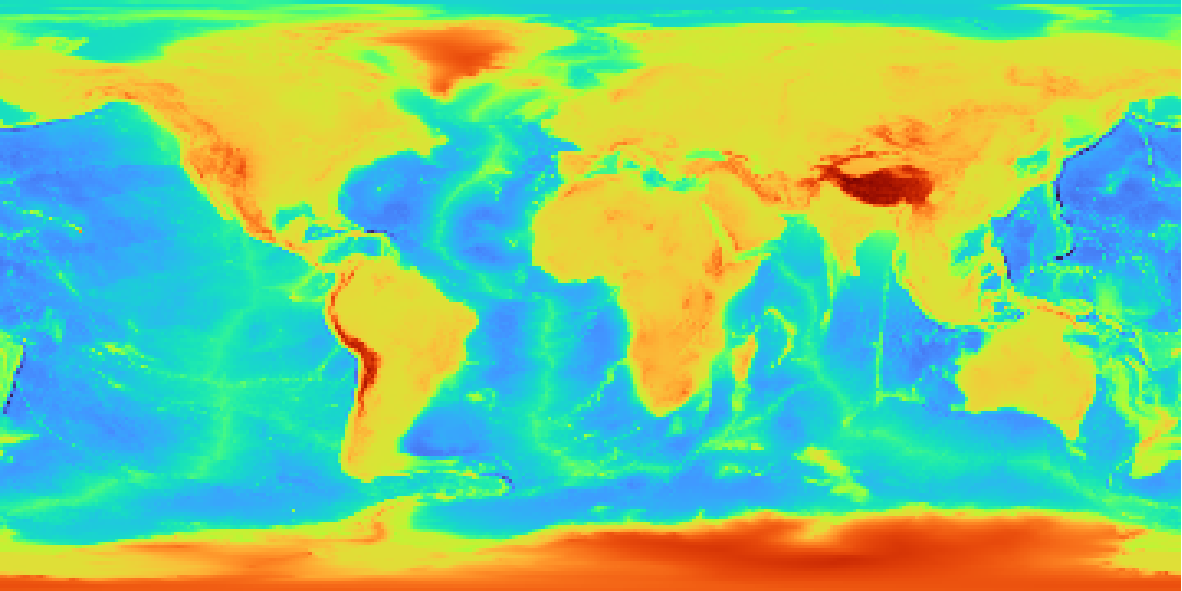Out:

```<IPython.core.display.Image object>
```

`pygmt.Figure.grdimage` can take the optional argument `projection` for the map. In the example below, the `projection` is set as `R12c` for 12 centimeter figure with a Winkel Tripel projection. For a list of available projections, see https://docs.generic-mapping-tools.org/latest/cookbook/map-projections.html.

```fig = pygmt.Figure()
fig.grdimage(grid=grid, projection="R12c")
fig.show()
```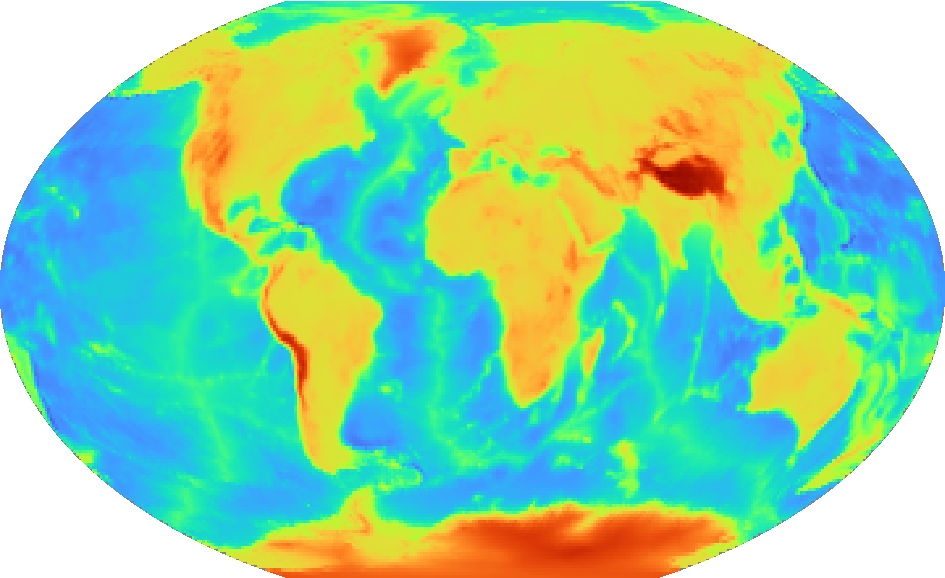Out:

```<IPython.core.display.Image object>
```

## Set a color map¶

`pygmt.Figure.grdimage` takes the `cmap` argument to set the CPT of the figure. Examples of common CPTs for Earth relief are shown below. A full list of CPTs can be found at https://docs.generic-mapping-tools.org/latest/cookbook/cpts.html.

Using the geo CPT:

```fig = pygmt.Figure()
fig.grdimage(grid=grid, projection="R12c", cmap="geo")
fig.show()
```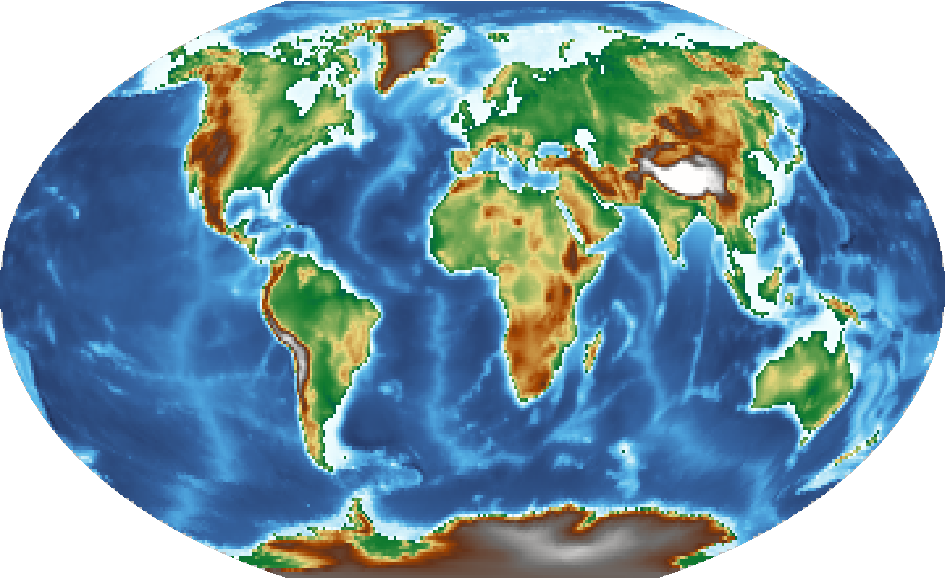Out:

```<IPython.core.display.Image object>
```

Using the relief CPT:

```fig = pygmt.Figure()
fig.grdimage(grid=grid, projection="R12c", cmap="relief")
fig.show()
```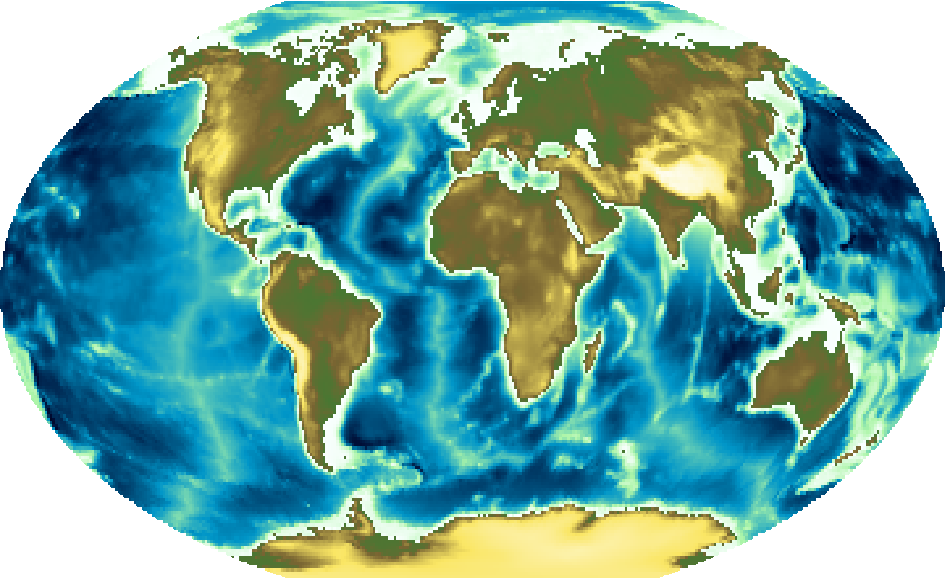Out:

```<IPython.core.display.Image object>
```

The `pygmt.Figure.colorbar` method displays the CPT and the associated Z-values of the figure, and by default uses the same CPT set by the `cmap` argument for `pygmt.Figure.grdimage`. The `frame` argument for `pygmt.Figure.colorbar` can be used to set the axis intervals and labels. A list is used to pass multiple arguments to `frame`. In the example below, `a2500` sets the axis interval to 2,500, `x+lElevation` sets the x-axis label, and `y+lm` sets the y-axis label.

```fig = pygmt.Figure()
fig.grdimage(grid=grid, projection="R12c", cmap="geo")
fig.colorbar(frame=["a2500", "x+lElevation", "y+lm"])
fig.show()
```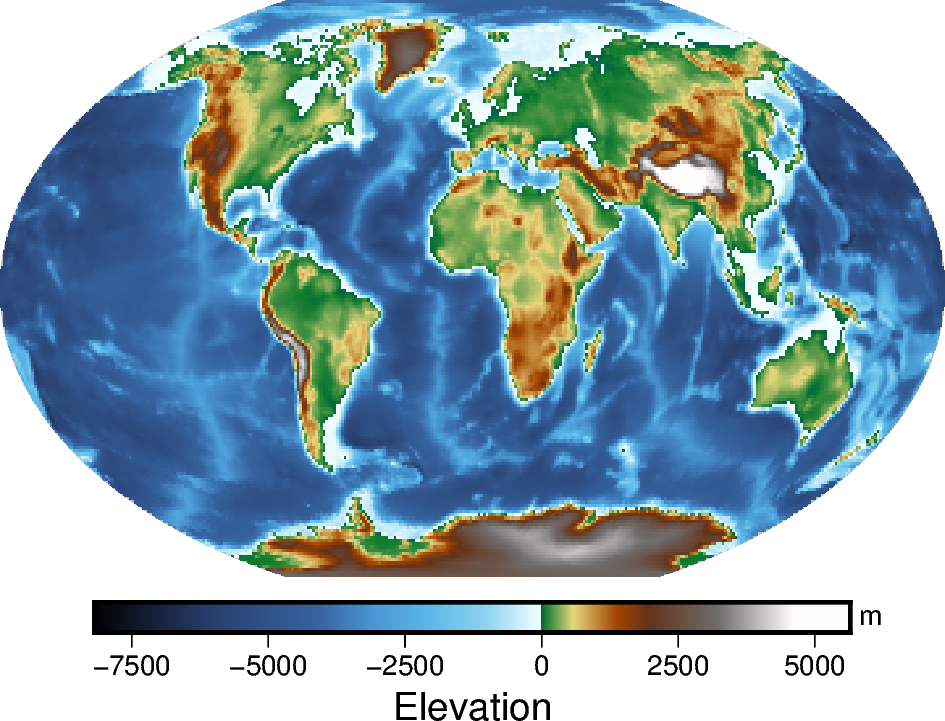Out:

```<IPython.core.display.Image object>
```

## Create a region map¶

In addition to providing global data, the `region` argument for `pygmt.datasets.load_earth_relief` can be used to provide data for a specific area. The `region` argument is required for resolutions at 5 arc minutes or higher, and accepts a list (as in the example below) or a string. The geographic ranges are passed as xmin/xmax/ymin/ymax.

The example below uses data with a 10 arc minute resolution, and plots it on a 15 centimeter figure with a Mercator projection and a CPT set to geo. `frame="a"` is used to add a frame to the figure.

```grid = pygmt.datasets.load_earth_relief(resolution="10m", region=[-14, 30, 35, 60])
fig = pygmt.Figure()
fig.grdimage(grid=grid, projection="M15c", frame="a", cmap="geo")
fig.colorbar(frame=["a1000", "x+lElevation", "y+lm"])
fig.show()
```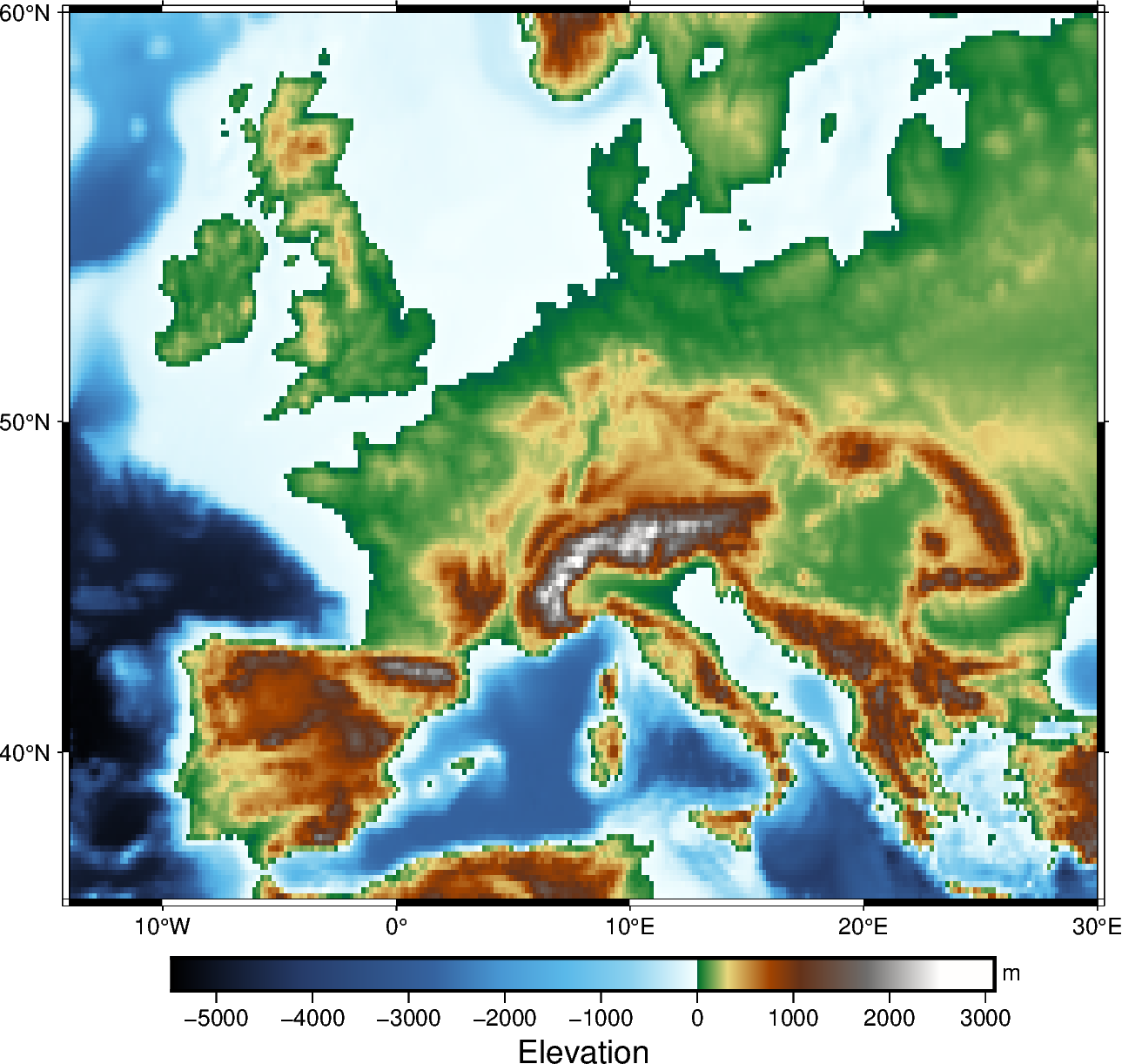Out:

```<IPython.core.display.Image object>
```

Total running time of the script: ( 0 minutes 5.974 seconds)

Gallery generated by Sphinx-Gallery